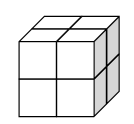### Home > CCG > Chapter 8 > Lesson 8.3.2 > Problem8-109

8-109.Reynaldo has a stack of blocks on his desk, as shown at right.

1. If his stack is $2$ blocks wide, $2$ blocks long, and $2$ blocks tall, how many blocks are in his stack?

(width)(length)(height) = the number of blocks in each stack

2. What if his stack instead is $3$ blocks wide, $3$ blocks long, and $2$ blocks tall? How many blocks are in this stack?

See part (a) for help.

3. What if his stack contains $99$ blocks, is $3$ blocks tall, $x$ blocks long, and $y$ blocks wide? What could $x$ and $y$ be?

Use the equation in part (a).
Substitute $99$ into the number of blocks and $3$ into the height.
What would $x$ and $y$ have to be in order to make this equation true?

Use the eTool below model the stacks of blocks for each part.
Click the link at right for the full version of the eTool: 8-109 HW eTool